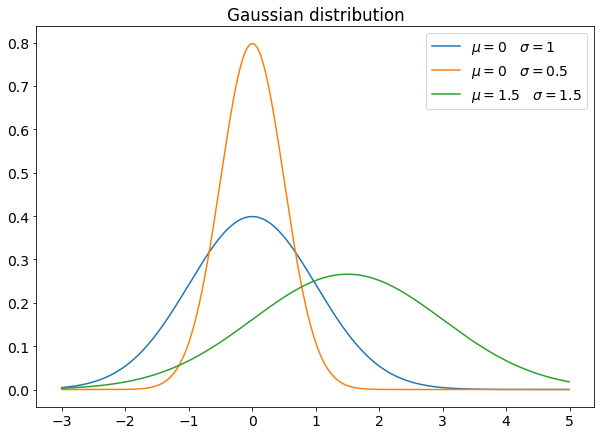# The most used probability distributions in Data Science

Original article was published on Artificial Intelligence on Medium# Uniform distribution

The simplest probability distribution is the uniform distribution, which gives the same probability to any points of a set.

In its continuous form, a uniform distribution between a and b has this density function: